• R语言可视化：散点图、散点图和折线图（line charts）、3D散点图、旋转3D散点图、气泡图、corrgram包可视化...
多人点赞
2022-01-13 17:11:07

# R语言可视化：散点图、散点图和折线图（line charts）、3D散点图、旋转3D散点图、气泡图、corrgram包可视化相关性矩阵、马赛克图（ Mosaic plots）、hexbin、密度图

目录r语言 数据挖掘 人工智能 机器学习
更多相关内容
• 主要介绍了matplotlib在python上绘制3D散点图实例详解，首先介绍了官网的实例，然后分享了本文简单代码示例，具有一定借鉴价值，需要的朋友可以了解下。
• 绘制了笛卡尔数据集的 3D 散点图。 数据点按颜色排序，并为映射到相同颜色的每组点调用一次 plot3。 这大大减少了大型数据集的执行时间。 默认情况下，这些点根据它们与 XY 平面的距离进行着色。 可以使用'ColorData...matlab
• python 绘图---2D、3D散点图折线图、曲面图，可以方便的进行科研绘图，学习交流
• 在一个三维座标系下画三维折线图，，，，，，，，，，three
• 3d 散点图 具有数据导入界面的 3D 散点图可视化JavaScript
• Matplotlib 是一个 Python 的 2D绘图库，它以各种硬拷贝格式和跨平台的交互式环境生成出版质量级别的图形，通过 Matplotlib，开发者可以仅需要几行代码，便可以生成绘图，直方图，功率谱，条形图，错误图，散点图等...

python中绘制2D曲线图需要使用到Matplotlib，Matplotlib 是一个 Python 的 2D绘图库，它以各种硬拷贝格式和跨平台的交互式环境生成出版质量级别的图形，通过 Matplotlib，开发者可以仅需要几行代码，便可以生成绘图，直方图，功率谱，条形图，错误图，散点图等。

## 1. 绘制折线图

plt.plot() 可以用于绘制折线图。只传入一维的散点(n个)p1时，横坐标对应散点的次序，从0到n-1，纵坐标对应散点的值。示例：

# -*- coding: utf-8 -*-
import matplotlib.pyplot as plt
import numpy as np

p1=[0,1.1,1.8,3.1,4.0]  # 数据点

#创建绘图图表对象，可以不显式创建，跟cv2中的cv2.namedWindow()用法差不多
plt.figure('Draw')

plt.plot(p1)  # plot绘制折线图

plt.draw()  # 显示绘图

plt.pause(5)  #显示5秒

plt.savefig("easyplot01.jpg")  #保存图象

plt.close()   #关闭图表

绘图：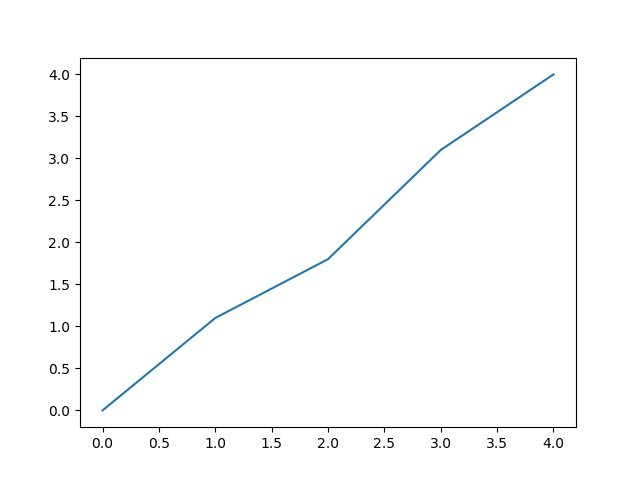plt.plot() 传入二维的散点p1,p2（p1和p2的长度要一样）时，横坐标x绘制p1,纵坐标y绘制p2，示例：

# -*- coding: utf-8 -*-
import matplotlib.pyplot as plt
import numpy as np

p1=[0,1.1,1.8,3.1,4.0]  # 数据点
p2=[2,2.4,4.3,3.5,2.5]

#创建绘图图表对象，可以不显式创建，跟cv2中的cv2.namedWindow()用法差不多
plt.figure('Draw')

plt.plot(p1,p2)  # plot绘制折线图

plt.draw()  # 显示绘图

plt.pause(5)  #显示5秒

plt.savefig("easyplot01.jpg")  #保存图象

plt.close()   #关闭图表

绘图：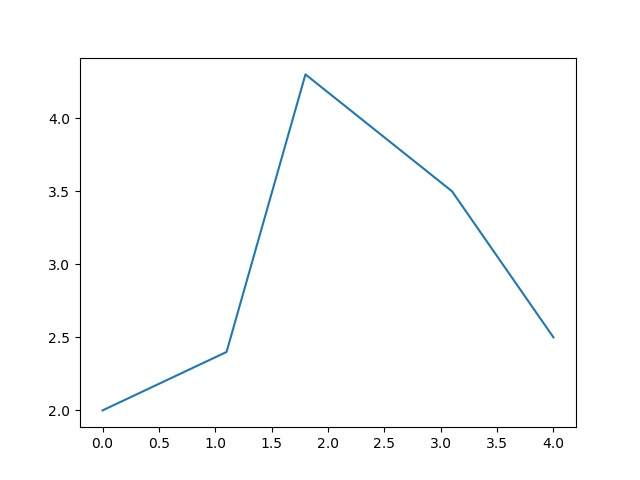## 2. 绘制散点图

plt.scatter()用于绘制散点图，传入参数必须是二维的：plt.scatter(p1,p2)，示例：

# -*- coding: utf-8 -*-
import matplotlib.pyplot as plt
import numpy as np

p1=[0,1.1,1.8,3.1,4.0]  # 数据点
p2=[2,2.4,4.3,3.5,2.5]

#创建绘图图表对象，可以不显式创建，跟cv2中的cv2.namedWindow()用法差不多
plt.figure('Draw')

plt.scatter(p1,p2)  # scatter绘制散点图

plt.draw()  # 显示绘图

plt.pause(10)  #显示10秒

plt.savefig("easyplot.jpg")  #保存图象

plt.close()   #关闭图表

绘图：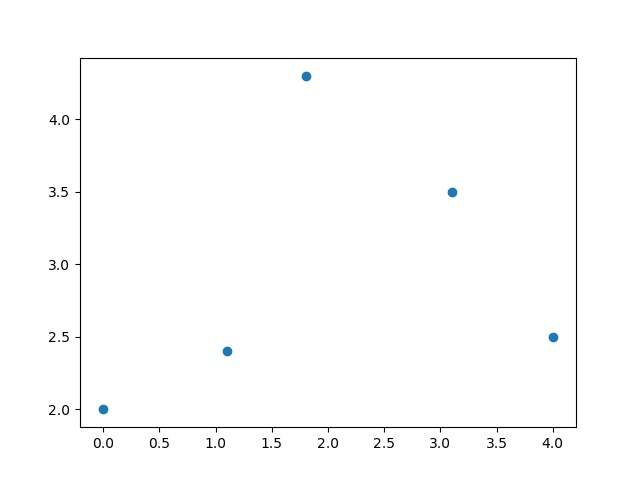plt.plot() 也可以用于绘制散点图，plt.plot(p1,p2,'ro')表示散点的颜色是红色，形状是o，示例：

# -*- coding: utf-8 -*-
import matplotlib.pyplot as plt
import numpy as np

p1=[0,1.1,1.8,3.1,4.0]  # 数据点
p2=[2,2.4,4.3,3.5,2.5]

#创建绘图图表对象，可以不显式创建，跟cv2中的cv2.namedWindow()用法差不多
plt.figure('Draw')

plt.plot(p1,p2,'ro')

plt.draw()  # 显示绘图

plt.pause(10)  #显示10秒

plt.savefig("easyplot.jpg")  #保存图象

plt.close()   #关闭图表

绘图：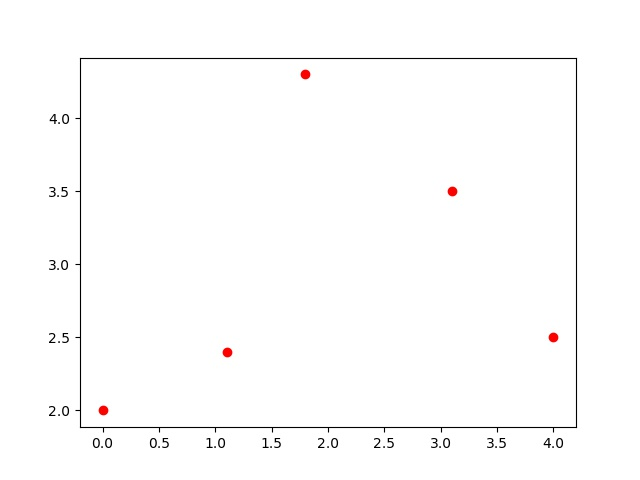## 3. 添加坐标轴说明、添加标题、图片中插入文字、显示网格

坐标轴说明：plt.xlabel()、plt.ylabel()
添加标题： plt.title()
插入文字： plt.text(60, .025, r'$number=5, sigma=0$')
显示网格： plt.grid(True)

# -*- coding: utf-8 -*-
import matplotlib.pyplot as plt
import numpy as np

p1=[0,1.1,1.8,3.1,4.0]  # 数据点
p2=[2,2.4,4.3,3.5,2.5]

#创建绘图图表对象，可以不显式创建，跟cv2中的cv2.namedWindow()用法差不多
plt.figure('Draw')

plt.plot(p1,p2,'ro')

plt.xlabel('number of samples')
plt.ylabel('value of samples')

plt.title('sample data')

plt.text(1.1, 3.6, r'$number=5, sigma=0$')

plt.grid(True)

plt.draw()  # 显示绘图

plt.pause(10)  #显示10秒

plt.savefig("easyplot.jpg")  #保存图象

plt.close()   #关闭图表

绘图：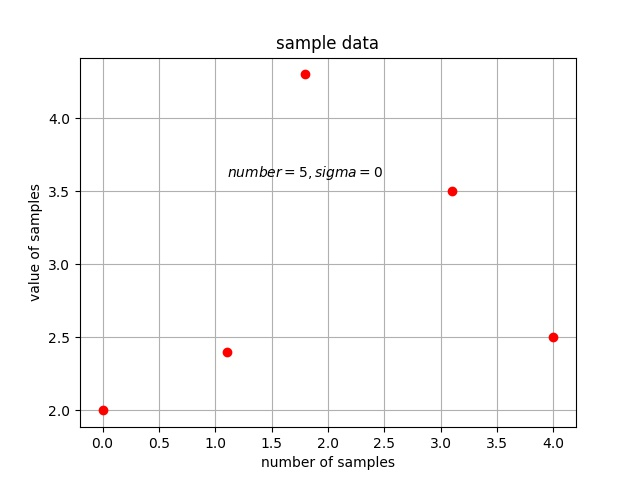## 4. 绘制3D图曲面图

plot_surface()用于绘制3D曲面图：

# -*- coding: utf-8 -*-
from matplotlib import pyplot as plt
import numpy as np
from mpl_toolkits.mplot3d import Axes3D

fig = plt.figure()
ax = Axes3D(fig)

X = [0,1.1,1.8,3.1,4.0]
Y = [2,2.4,4.3,3.5,2.5]
X, Y = np.meshgrid(X, Y)
Z = np.array([[2,2.4,4.3,3.5,2.5]])

ax.plot_surface(X, Y, Z, rstride=1, cstride=1, cmap='rainbow')

plt.draw()
plt.pause(10)
plt.savefig('3D.jpg')
plt.close()


绘图：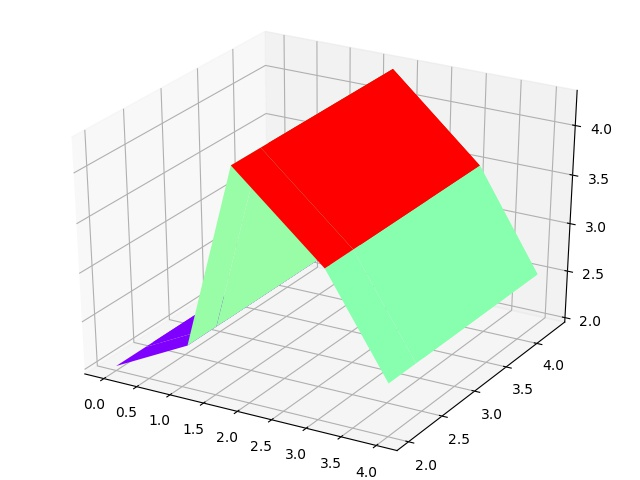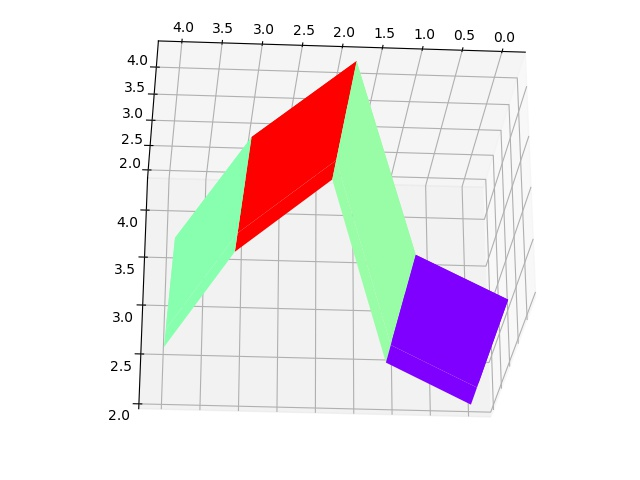使用plot_surface()绘制另一个3D曲面：

# -*- coding: utf-8 -*-
from matplotlib import pyplot as plt
import numpy as np
from mpl_toolkits.mplot3d import Axes3D

fig = plt.figure()
ax = Axes3D(fig)
X = np.arange(-4, 4, 0.25)
Y = np.arange(-4, 4, 0.25)
X, Y = np.meshgrid(X, Y)
R = np.sqrt(X**2 + Y**2)
Z = X*Y

ax.plot_surface(X, Y, Z, rstride=1, cstride=1, cmap='rainbow')

plt.draw()
plt.pause(10)
plt.savefig('3D.jpg')
plt.close()

绘图：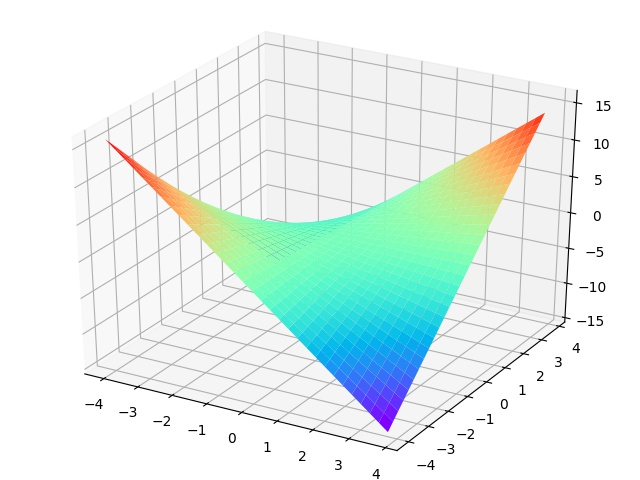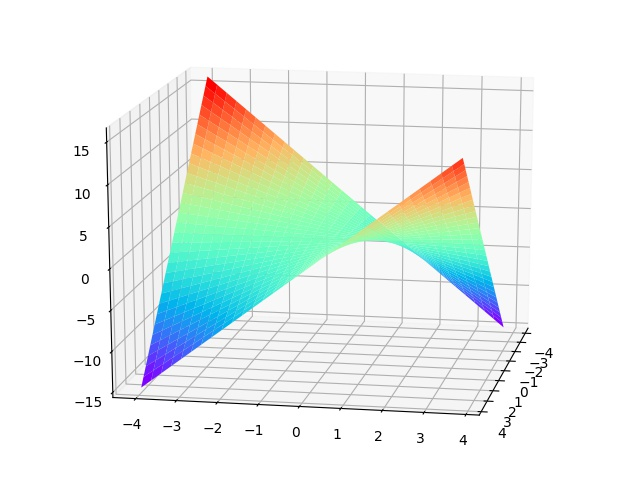## 5. 绘制3D散点图

scatter()用于绘制3D散点图：

# -*- coding: utf-8 -*-
import numpy as np
import matplotlib.pyplot as plt
from mpl_toolkits.mplot3d import Axes3D

x = [0,1.1,1.8,3.1,4.0]
y = [2,2.4,4.3,3.5,2.5]
z = [1,2,3,4,5]

ax = plt.subplot(projection='3d')  # 创建一个三维的绘图工程

ax.scatter(x, y, z, c='r')  # 绘制数据点,颜色是红色

ax.set_zlabel('Z')  # 坐标轴
ax.set_ylabel('Y')
ax.set_xlabel('X')

plt.draw()
plt.pause(10)
plt.savefig('3D.jpg')
plt.close()

绘图：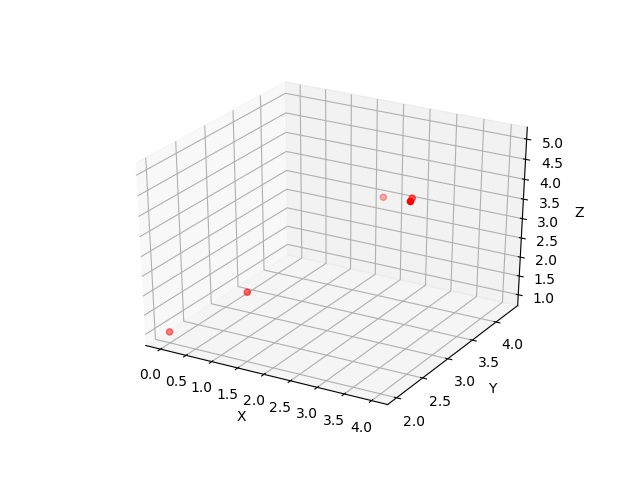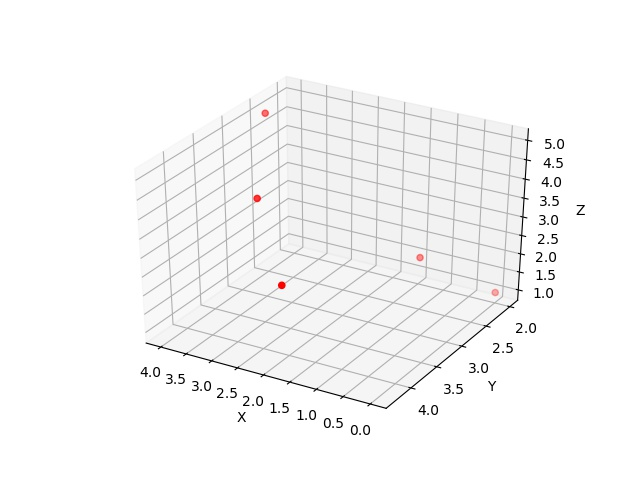展开全文scatter plt.plot
• 一、3D折线图 import numpy as np import matplotlib.pyplot as plt from mpl_toolkits.mplot3d import Axes3D import random import matplotlib as mpl from matplotlib import cm from mpl_toolkits.mplot3d ...
公众号: 数据小斑马,关注即可获得价值1000元的数据分析学习资料


## 一、3D折线图

公众号: 数据小斑马,关注即可获得价值1000元的数据分析学习资料

import numpy as np
import matplotlib.pyplot as plt
from mpl_toolkits.mplot3d import Axes3D
import random
import matplotlib as mpl
from matplotlib import cm
from mpl_toolkits.mplot3d import axes3d
from mpl_toolkits.mplot3d.art3d import Poly3DCollection,Line3DCollection

x = np.linspace(1,20,20)
y = np.arange(10,30,1)
z = np.random.randint(20,50,20)  # numpy分别生成三个维度数据
fig = plt.figure()
ax.plot(x,y,z,'bo--')  # 参数与二维折现图不同的在于多了一个Z轴的数据
plt.show()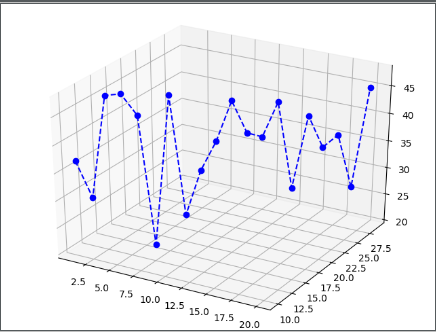## 二、3D曲面图

公众号: 数据小斑马,关注即可获得价值1000元的数据分析学习资料

data = np.random.randint(100,500,(3,10,20))
x,y,z = data,data,data  # numpy同时生成三维数据
fig = plt.figure()
ax1.plot_surface(x,y,z,cmap=plt.cm.winter,rstride=1,cstride=1) # rstride和cstride是隔几行几列取一个数字，代表曲面的稀疏度
ax2.plot_surface(x,y,z,cmap=plt.cm.winter,rstride=10,cstride=10)
plt.show()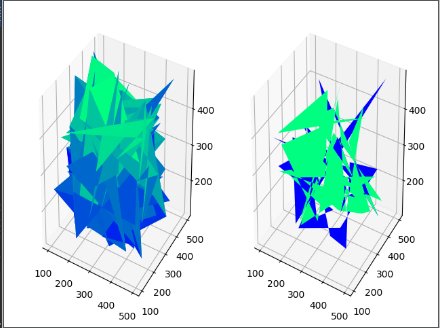## 三、3D散点图

公众号: 数据小斑马,关注即可获得价值1000元的数据分析学习资料

x= np.linspace(5,20,20)
y = np.linspace(20,100,20)
x1,y1 = np.meshgrid(x,y)
z = np.sin(x1)*y1+np.sin(y1)*x1  # 函数构造数据
fig = plt.figure()
ax1.scatter(x1,y1,z,c='y',marker='D')
ax2.scatter(x1[:10],y1[:10],z[:10],cmap=plt.cm.winter,marker='o')
ax2.scatter(x1[10:],y1[10:],z[10:],cmap=plt.cm.spring,marker='*')
plt.show()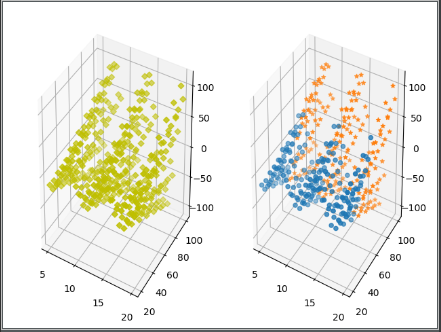四、等高线图

x = np.arange(-10,10,0.01)  #生成步长为0.01的数据
y = np.arange(-10,10,0.01)
x,y=np.meshgrid(x,y)
def func(x,y):
return x**2+y**2
fig,axes = plt.subplots(2,2)
axes[0,0].contour(x,y,func(x,y),20,cmap=plt.cm.winter,alpha=0.8) # 不填充，只是线20是指分成20等份，分太多会看不清
axes[0,1].contourf(x,y,func(x,y),20,cmap=plt.cm.hot)  # 填充
c = axes[1,0].contour(x,y,func(x,y),[8,20],c='k')  # [8,20]是只想看这两条线
plt.clabel(c,inline=True,fontsize=10,fmt='%.f',colors=['k','y']) # 设置数据标签格式，inline是在线上
d = axes[1,1].contourf(x,y,func(x,y),10,cmap=plt.cm.hot)
plt.clabel(d,inline=True,fontsizq=10,fmt='%.f')
plt.colorbar(d) # 显示数据条
plt.xticks(())
plt.yticks(()) # 去除坐标轴
plt.show()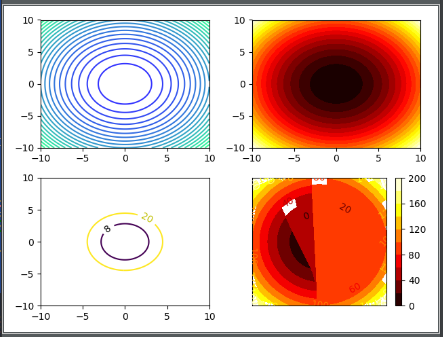本人互联网数据分析师，目前已出ExcelSQLPandasMatplotlibSeaborn机器学习统计学个性推荐关联算法工作总结系列。

微信搜索 " 数据小斑马" 公众号
1、回复“数据分析"就可以免费领取数据分析升级打怪 15本必备教材展开全文等高线图
• 除了常见的二维图表，在文献中也会见到3维的图表，如下图的PCA分析图表。...图表绘制数据整理后，通过 Plot/ 3D Scatter (如下图)绘制3D散点图。在数据选择窗口，A、B、C三列数据分别对应X、Y、Z，点击OK...

除了常见的二维图表，在文献中也会见到3维的图表，如下图的PCA分析图表。数据准备

示例所用的数据如下，假设A、B、C三列数据为3个主成分，D列为样本。绘制3D的图表需要Z轴，因此我们需要将一列数据转换为“Z”，方法如下图，我们这里将C列数据转化为“Z”。图表绘制

数据整理后，通过 Plot/ 3D Scatter (如下图)绘制3D散点图。在数据选择窗口，A、B、C三列数据分别对应X、Y、Z，点击OK。立即可获得初步的绘制结果，见下图，单击图表内的空白区域，可对图表进行平移、缩放和旋转。右键图表中的“小球球”，在菜单中点Plot Details(如下图)，可对这些“球球”进行进一步的调整，如大小、颜色、形状(不仅仅是球形，你也可以选择正方体、星形等等)的改变等。除了上面这些，当然比较重要的是要建立“颜色索引”，我想实现给3个样本分别进行着色。方法如下图，点击Color 右侧的小三角，在By Points选项卡，将D列的“Species”数据作为颜色的Indexing。这样，小球球就按D列的数据分成三种颜色啦。嗯，图例还有问题，接下来我们需要让PC1和PC2也显示出来。右键图表中的图例，通过Legend\Reconstruct Legend(如下图) 让PC1和PC2也显示出来。图表调整

关于3D图表的调整如坐标轴的调整与之前讲的2D图表的调整一致，如果有轴标签出现重叠的现象，可通过对坐标轴的Scale进行微调来“错开”，你也可用3D Rotation工具(如下图，窗口左侧工具栏也有)对图表进行旋转、倾斜、改变透视关系等，非常方便，大家可以试一试。最终的导出结果如下图：展开全文• Echarts3D散点图 下载 npm install echarts-gl <template> <div :id="id"></div> </template> <script> import * as echarts from "echarts"; import "echarts-gl"; export ...echarts
•javascript 数据可视化
• 准备工作： 1.npm install echarts@4.1.0 --save ...import'echarts-gl'//制作3D图数据可视化相关图表时需引入 import'echarts/lib/component/visualMap'//按需引入 Vue.prototype.\$echarts=echarts 3.页面效果...echarts css html
• Python 用 matplotlib 绘制 3D 散点图 import numpy as np import matplotlib.pyplot as plt import mpl_toolkits.mplot3d from mpl_toolkits.mplot3d import Axes3D m= np.array([[1,3,3], [4,1,6], [1,4,2]...python
• 通过ax.scatter3D()来使用Matplotlib的mplot3d工具包，创建一个3D散点图 示例1： # Import libraries from mpl_toolkits import mplot3d import numpy as np import matplotlib.pyplot as plt # Creating ...matplotlib python
• x = np.linspace(0, 1, 100) y = np.sin(x * 2 * np.pi) / 2 + 0.5 ax.plot(x, y, zs=0, zdir='z', label='curve in (x,y)') # 在X和Z轴上绘制散点图数据（每种颜色20个点）。 colors = ('r', 'g', 'b', 'k') np....python
• 这是一个如何在MATLAB:registered:中创建3D散点图的示例。 在MATLAB文档中阅读有关“ scatter3”函数的信息。 有关更多示例，请转到 MATLAB 绘图库 - http://www.mathworks.com/discovery/gallery.htmlmatlab
• ## echarts 3D折线图应用

千次阅读 2020-07-13 13:25:09
公司最近做一个振动项目，需要展示振动曲线，考虑其直观性，决定用3D折线图来做。 首先你要引入echarts的相关js，下图为我引入的例子，其中GL那个js是echarts控制3D的，你可根据自己需要去echarts官方网站上下载这俩...html
• #在pyecharts中怎么才能实现下面在3d散点图中的标记呢？(下面是效果图)，百思不得其解...有问必答 python
• SCATTER3SPH 就像 scatter3 只绘制球体，而不是平面圆。 对于两个变量（X 和 Y）的每个组合，它可以表示三个量：高度 (Z)、颜色和大小。 无论轴缩放如何，球体看起来都是“球形”。 SCATTER3SPH(X,Y,Z) 在向量 X、Y ...matlab
• 效果很好的2D饼图、2D柱状图、3D饼图、3D柱状图、3维2D折线图、3维2D柱状图、3维3D柱状图制作源码，操作简单，只要将js文件拷到项目中即可，适用于很多图形报表开发。
• matplotlib中提供的是比较基本的3D散点图功能，并未做特别的强化。 在单独窗口中可以做三维旋转观察，此外并无更多功能。 Axes3D.scatter( xs, ys : 散点的x/y坐标 zs = 0 : 散点的z坐标，默认为0 zdir = ‘z’ : 当...
• set.seed(417) library(plotly) temp (100, mean=30, sd=5) pressure (100) dtime <- 1:100 Graphing your 3d scatterplot using plotly's scatter3d type: plot_ly(x=temp, y=pressure, z=dtime, type="scatter3d...
• from pyecharts.charts import Scatter3D if __name__ == '__main__': a = np.arange(1, 10, 0.1) b = a * 2 + 10 c = a + b # 这里的 data 格式是 [ (x,y,z), (x,y,z), (x,y,z), ........] data = lipython
• 数据可视化_3D散点图_论文插图_Python代码实现()
• <div style="border: 2px solid #000;width: 100%;height: 100%;"> <div id="main" style="border: 1px solid green;width: 100%;height: 100%;float: left;background: #999;"><....echarts
• 这是一个易于使用的3D散点图功能。 与八度高度兼容。matlab
• ## python 绘制3D散点图

千次阅读 2020-07-02 10:26:19
绘制3D散点图 """ 绘制三维散点图 """ import numpy as np import matplotlib.pyplot as mp from mpl_toolkits.mplot3d import axes3d # 1.生成数据 n = 200 x = np.random.normal(0, 1, n) y = np.random.normal...
• Python生成3D图，饼图，合图，散点图折线图，柱状图
• 借鉴在Matplotlib文档页面上的example，并稍微修改代码，import numpy as npfrom mpl_toolkits.mplot3d import Axes3Dimport matplotlib.pyplot as pltdef randrange(n, vmin, vmax):return (vmax-vmin)*np.random.......